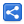# Groovy Operators - Power Operator

[Last Updated: Dec 5, 2018]

In following tutorial we will quickly go through all the operators which both Java and Groovy support.

We will also understand Groovy specific power operator.

## Arithmetic operators

Groovy supports all Java arithmetic operators i.e. +, -, *, / and %.

### The power operator

Additionally Groovy includes a new operator ** in the list. this operator is used to perform power operation.

`println 2**4`
`16`

As Groovy converts primitives to corresponding Number wrappers (tutorial), the resultant value of all above operators will also be also one of the Number type:

```def x = 2 * 2.1 //groovy always converts decimal number to bigDecimal
println x
println x.getClass()
`4.2class java.math.BigDecimal7.2`
```def x = 2.1 ** 2
println x
println x.getClass()
println x.toBigInteger()```
`4.41class java.math.BigDecimal4`

## Unary operators

All Java unary operators are supported:

```+    for positive value of the operand, -    for negative value of the operand,
++   increment, postfix/prefix,--   decrement, post/prefix and !    logical complement operator ```

## Assignment arithmetic operators

All assignment operators of Java are supported in the same way. These operators are =, +=, -=, *=, /= and %=

Additionally Groovy also has assignment operator for its power operation i.e. **=

```def x = 5
x **= 3// same as x = x**3
println x```
`125`

## Equality and Rational operator

Same as Java:
== (equal), != (not equal), < (less than), <= (less than or equal), > (greater than) and >= (greater than or equal).

## Rational operators (Conditional operators)

Same as Java:
&& (logical and), || (logical or) and ! (logical not).
&& and || also exhibit 'short-circuiting behavior).

## Bitwise operators

Same as Java:
& (bitwise and), | (bitwise or), ^ (bitwise xor) and ~ (bitwise negation).

## Ternary operator

Same as Java:
result = boolean expression? result1: result2

 Share